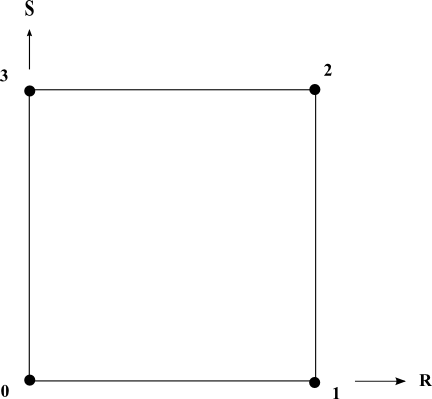• ```public class MxQuadrangleCellExtract
extends MxCellExtract```

Utility class that provides a static implementation of `MiSurfaceCell` 's methods for a quadrangle cell. This class is provided to make easier the creation of a class that implements the `MiSurfaceCell` interface for a quadrangle cell.

Localization test
See `isPointInsideCell(MiGeometryI, MiSurfaceCell, double[], double[])`

The following image shows the node numbering and the face numbering used by this class.

Nodes and faces numbering• ### Method Summary

All Methods
Modifier and Type Method Description
`static double` ```getLongestEdgeLength​(MiGeometryI meshGeometry, MiSurfaceCell cell)```
Gets the longest edge of a quadrangle cell.
`static boolean` ```isPointInsideCell​(MiGeometryI meshGeometry, MiSurfaceCell cell, double[] point, double[] weights)```
Checks if a point is inside or outside a quadrangle cell.
• ### Methods inherited from class com.openinventor.meshvizxlm.extractors.cell.MxCellExtract

`getCenter`
• ### Methods inherited from class java.lang.Object

`equals, getClass, hashCode, notify, notifyAll, toString, wait, wait, wait`
• ### Method Detail

• #### getLongestEdgeLength

```public static double getLongestEdgeLength​(MiGeometryI meshGeometry,
MiSurfaceCell cell)```
Gets the longest edge of a quadrangle cell.
Parameters:
`meshGeometry` - the geometry of the mesh
`cell` - the input cell
Returns:
the longest edge of the specified cell
• #### isPointInsideCell

```public static boolean isPointInsideCell​(MiGeometryI meshGeometry,
MiSurfaceCell cell,
double[] point,
double[] weights)```
Checks if a point is inside or outside a quadrangle cell.

This static method helps to implement the method `MiCell.isPointInsideCell(MiGeometryI, double[], double[])` for a quadrangle cell.

Parameters:
`meshGeometry` - the geometry of the mesh
`cell` - the input cell.
`point` - the input point given in the same space coordinate as meshGeometry
`weights` - the array into which the weight values are to be stored. This method computes the 4 values weights[0-3] if the point is inside the cell. It assumes the weights array has been already allocated. Its size must be set to 4 (at least) before calling this method
Returns:
`true` if the point is inside the cell